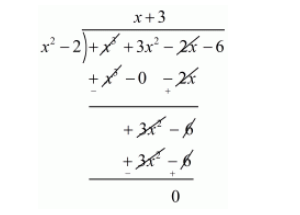# Find all the zeros of the polynomial x3 + 3x2 − 2x − 6,

Question:

Find all the zeros of the polynomial $x^{3}+3 x^{2}-2 x-6$, if two of its zeros are $-\sqrt{2}$ and $\sqrt{2}$.

Solution:

We know that if $x=\alpha$ is a zero of a polynomial, and then $x-\alpha$ is a factor of $f(x)$.

Since $\sqrt{2}$ and $-\sqrt{2}$ are zeros of $f(x)$.

Therefore

$(x+\sqrt{2})(x-\sqrt{2})=x^{2}-(\sqrt{2})^{2}$

$=x^{2}-2$

$x^{2}-2$ is a factor of $f(x)$.Now, we divide $x^{3}+3 x^{2}-2 x-6$ by $g(x)=x^{2}-2$ to find the other zeros of $f(x)$.By using division algorithm we have $f(x)=g(x) \times q(x)-r(x)$

$x^{3}+3 x^{2}-2 x-6=\left(x^{2}-2\right)(x+3)-0$

$=(x+\sqrt{2})(x-\sqrt{2})(x+3)$

Hence, the zeros of the given polynomials are $-\sqrt{2},+\sqrt{2}$, and $-3$.